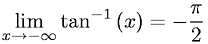Equations > Calculus > Limits > Limit of Arctangent X as X Approaches Negative Infinity

### Limit of Arctangent X as X Approaches Negative InfinityLatex Code:

MathML Code:

 $\underset{x\to -\infty }{lim}{\mathrm{tan}}^{-1}\left(x\right)=-\frac\pi 2$

MathType 5.0: